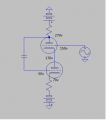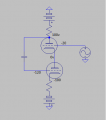# Relative voltages question.

#### coinmaster

Joined Dec 24, 2015
502
I have a question about relative voltages and operating points of components, in this case tubes.
Are these two functionally the same?For a while now I never could understand how the bottom triode of the WCF in an amp I bought was biased at -100v on the grid and 0 volt on the plate which would put the tube way into cutoff. It just dawned on me that since the cathode is -75v then 0v is effectively +75v on the load line for the triode and -100v is actually -20v.

It then occurred to me that if 0v = +75v as far as the triode is concerned then can high positive voltages relative to other parts of the amplifier be considered low voltages in the WCF? For example the picture on the left, to each triode the relative voltages are the same as the voltages on the right.
Does this mean that they would both function the same? In this case is 170v on the output of the left picture effectively the same as 0v to a speaker I hook up to it? (Assuming the other end of the speaker is also 170V)
Or perhaps if I connected the picture on the left directly to a 150v input stage would it still be considered the same as the picture on the right as far as the tubes are concerned?

Last edited:

#### wayneh

Joined Sep 9, 2010
17,271
I can't follow every detail of your drawing and question, but yes, all voltages are relative. If you have a working schematic and then subtract 200V from every voltage on the schematic, the circuit will be be identical. But that applies to circuit "ground" as well – you have to adjust ALL the voltages to maintain the same ∆V seen by every component.

#### RichardO

Joined May 4, 2013
2,270
Both diagrams look like they would operate the same.

This is a common technique in oscilloscope CRT circuits. The deflection plates are biased near ground so that low voltage is on the output amplifier. The anode has a very high positive voltage and cathode is biased with a large negative voltage.

#### ErnieM

Joined Apr 24, 2011
8,355
Since neither schematic indicated to where these voltages are referenced the only thing that can be said is the difference between points is the same.

Note I have a further assumption wrapped up there that each circuit's measurements reference the same point.